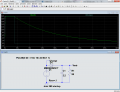# Finding current in a circuit with capacitors discharging to a DC Motor

#### t.nelson

Joined Feb 24, 2016
5
Hey all,

I currently have a circuit that charges 13 2.2 Farad Capacitors in parallel using a 1.5 V AA battery in 60 seconds. Then I flip some switches to put the capacitors in series and disconnect from the battery. This gives me a total voltage in the capacitor matrix of about 20 Volts where they are then going to discharge to this DC Motor: http://www.jameco.com/1/1/52249-ls5...amps-dc-motor-10823-rpm-25-4-c-gm-torque.html.

How would I go about analyzing this discharge circuit to find the current. I realize that a DC Motor has an inductor but don't know the other components or how these would affect the current. (the only thing I know is that it will change as time goes by)

Any help or being pointing in the right direction is extremely appreciated.

Thanks!

#### ronv

Joined Nov 12, 2008
3,770
Hey all,

I currently have a circuit that charges 13 2.2 Farad Capacitors in parallel using a 1.5 V AA battery in 60 seconds. Then I flip some switches to put the capacitors in series and disconnect from the battery. This gives me a total voltage in the capacitor matrix of about 20 Volts where they are then going to discharge to this DC Motor: http://www.jameco.com/1/1/52249-ls5...amps-dc-motor-10823-rpm-25-4-c-gm-torque.html.

How would I go about analyzing this discharge circuit to find the current. I realize that a DC Motor has an inductor but don't know the other components or how these would affect the current. (the only thing I know is that it will change as time goes by)

Any help or being pointing in the right direction is extremely appreciated.

Thanks!
Sounds simple - but it's not. There a a lot of variables such as efficiency that are hard to calculate as the voltage changes.
If you ignore all that and assume the motor is running at maximum efficiency it would run for about 2 minutes.Edit:

#### t.nelson

Joined Feb 24, 2016
5
Sounds simple - but it's not. There a a lot of variables such as efficiency that are hard to calculate as the voltage changes.
If you ignore all that and assume the motor is running at maximum efficiency it would run for about 2 minutes.
View attachment 101322
How would I calculate the time constant for the capacitor; would I still use t=RC?

#### ronv

Joined Nov 12, 2008
3,770
How would I calculate the time constant for the capacitor; would I still use t=RC?
That will at least give you a rough idea. That's what I did in the simulation was use a motor equivalent resistance of 58 ohms.

#### alfacliff

Joined Dec 13, 2013
2,458
12 2.2 mfd in series would be .169 farad. charge in paralell at 1.5 volt and discharge in series at starting voltage of aprox. 20 vds and .169 farad.

#### t.nelson

Joined Feb 24, 2016
5
Ok, I think I got it.

Thanks!

Joined Jul 18, 2013
26,254
Have you taken into consideration the generated voltage that is of the same polarity and opposes the supply voltage as the motor is running (BEMF)?
Of course this will also affect the current.
It is not the same a simple inductive load.
Max.

#### Alec_t

Joined Sep 17, 2013
13,224
If the motor is driving some mechanical load its current draw will be something between its free-running current and its stall current. So that's another complication.

#### ronv

Joined Nov 12, 2008
3,770
I gave you some bad numbers. I used 2.2F for the time before the cap was discharged. It's about an order of magnitude less than that when you hook them in series.

#### t.nelson

Joined Feb 24, 2016
5
Have you taken into consideration the generated voltage that is of the same polarity and opposes the supply voltage as the motor is running (BEMF)?
Of course this will also affect the current.
It is not the same a simple inductive load.
Max.
Is the back EMF large enough that I need to include it into my calculations, taking into account that I am already assuming the capacitors are theoretically perfect and that the resistance from the switches is negligible?

I already calculated the average current draw from the motor, at a given torque, as the capacitor decays to 12 volts. I then found the extra resistance I need to manipulate the time constant to the time I want.

I will also have a gearbox connected to the motor that I need to optimize. I used the calculus average value theorem to find the average voltage in my desired time period. Would it be accurate enough to use the stall torque and free speed constants of the motor at this average voltage?

#### t.nelson

Joined Feb 24, 2016
5
I gave you some bad numbers. I used 2.2F for the time before the cap was discharged. It's about an order of magnitude less than that when you hook them in series.
What would that do to the numbers you gave me?

Joined Jul 18, 2013
26,254
The BEMF should be included, but the load of a G.B. when power is removed usually results in a very fast decrease in rpm, depending on ratio, rather than a coast to stop condition.
Max.

#### ronv

Joined Nov 12, 2008
3,770
What would that do to the numbers you gave me?
If you look at the motor spec it draws .41 amps at 24 volts at it's most efficient point. So it looks like 58.5 ohms to the caps.
So yes, your right T=RC. so with 13 2.2F in series they look like .169F. This gives a time constant of about 10 seconds. So in about 10 seconds the voltage will be down to about 6 volts if you allow a little for start up. In real life it won't be that good because the motor won't be running at the best point for the whole time.